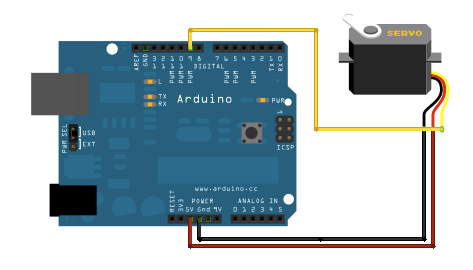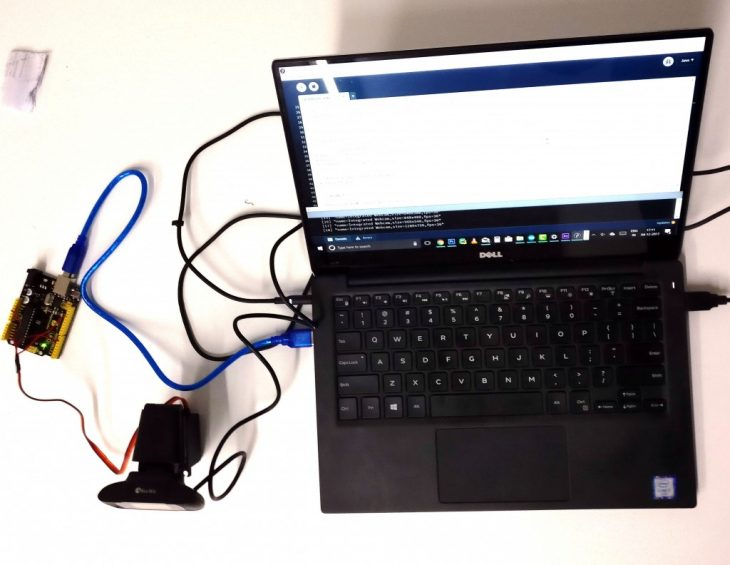## Program

### TrackCam : DIY Camera Tracking App

MAA 01

SO.4 - Produino

#### Tags

TrackCam is a project developed for SO.4 of MAA 2017-2018. The objective of this exercise is to develop a project that combines processing and Arduino.

TrackCam helps the user achieve automatic control over camera movement with the assigned subject being the focus of the camera. This is done using processing’s video library which reads the colour of each pixel through camera livefeed and isolates the movement of assigned colour to be tracked. This movement information is used to control the rotation of servo motor which holds the camera.

Components required :

• Arduino Uno
• Web-cam
• 360 degree servo motor
• Jumper wiresComponents requiredArduino – Servo Circuit DiagramConnected Setup

Arduino sketch used for this project is the “StandardFirmata” example sketch.

Processing sketch used for this project is

———————————————–

```import processing.serial.*;

import cc.arduino.*;

Arduino arduino;

import processing.video.*;
int servoPin = 6;
int speed = 90;
int delayTime = 50;

Capture video;

color trackColor;
float threshold = 25;

void setup() {

println(Arduino.list());
arduino = new Arduino(this, Arduino.list(), 57600);
arduino.pinMode(servoPin, Arduino.SERVO);

//  --------------------------------------------------

size(640, 480);
String[] cameras = Capture.list();
printArray(cameras);
video = new Capture(this, cameras);
video.start();
trackColor = color(255, 0, 0);
}

void captureEvent(Capture video) {
}

void draw() {

arduino.servoWrite(servoPin, speed);

image(video, 0, 0);

//threshold = map(mouseX, 0, width, 0, 100);
threshold = 10;

float avgX = 0;
float avgY = 0;

int count = 0;

for (int x = 0; x < video.width; x++ ) {
for (int y = 0; y < video.height; y++ ) {
int loc = x + y * video.width;

color currentColor = video.pixels[loc];
float r1 = red(currentColor);
float g1 = green(currentColor);
float b1 = blue(currentColor);
float r2 = red(trackColor);
float g2 = green(trackColor);
float b2 = blue(trackColor);

float d = distSq(r1, g1, b1, r2, g2, b2);

if (d < threshold*threshold) {
stroke(255);
strokeWeight(1);
point(x, y);
avgX += x;
avgY += y;
count++;
}
}
}

if (count > 0) {
avgX = avgX / count;
avgY = avgY / count;

fill(255);
strokeWeight(4.0);
stroke(0);
ellipse(avgX, avgY, 12, 12);
rectMode(CENTER);

if (avgX> 5*width/8 && avgX<width ) {
speed = 85;
arduino.servoWrite(servoPin, speed);
println(avgX);
delay(delayTime/2);
} if (avgX< 3*width/8 && avgX>0 ) {
speed = 95;
arduino.servoWrite(servoPin,speed);
println(avgX);
delay(delayTime);
} else {
speed = 90;
arduino.servoWrite(servoPin, speed);
println(avgX);
}
saveFrame();
}
}

float distSq(float x1, float y1, float z1, float x2, float y2, float z2) {
float d = (x2-x1)*(x2-x1) + (y2-y1)*(y2-y1) +(z2-z1)*(z2-z1);
return d;
}

void mousePressed() {
int loc = mouseX + mouseY*video.width;
trackColor = video.pixels[loc];
}```

——————————————————-

Studio SO.4  faculty :Angelos Chronis and Angel Munoz

TrackCam is a project of IaaC, Institute for Advanced Architecture of Catalonia

developed at MAA1 in 2017-2018 by:

Student: Aman Jain